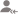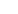true

Take Class 8 Tuition from the Best Tutors

•Affordable fees
•1-1 or Group class
•Flexible Timings
•Verified Tutors

Search in# Learn Mathematics with Free Lessons & Tips

Post a Lesson

All

All

Lessons

Discussion

Lesson Posted on 30/06/2019 Learn Mathematics +1I am MBA Finance. I believe in conceptual learning and not just theoretical

Understanding relationship between natural numbers, whole numbers and integers
Dislike Bookmark

Lesson Posted on 09/06/2019 Learn Mathematics +1I am MBA Finance. I believe in conceptual learning and not just theoretical

Please go through this video which will help you to know the conecpt of Natural numbers, Whole numbers and Integers.

Dislike Bookmark

Lesson Posted on 28/06/2017 Learn Mathematics +3I am an experienced, qualified teacher and tutor with over 20 years of experience in teaching Maths and...

We know, in unitary method, we first find the value of one quantity from the value of the given quantity. Then we use this value to find the value of the required quantity. While working out the problems using unitary method we come across certain variations in which the values of two quantities depend... read more

We know, in unitary method, we first find the value of one quantity from the value of the given quantity. Then we use this value to find the value of the required quantity. While working out the problems using unitary method we come across certain variations in which the values of two quantities depend on each other in such a way that a change in one, results in a corresponding change in the other; then the two quantities are said to be in variation and the two types of variation which occur are called direct and inverse variations.

Solved examples of mixed problems using unitary method:

1. If 24 painters working for 7 hours a day, for painting a house in 16 days. How many painters are required working for 8 hours a day will finish painting the same house in 12 days?

Solution:

24 painters working for 7 hours paint a house in 16 days.

1 painter working for 7 hours paints a house in 16 × 24 days.

1 painter working for 1 hour paints a house in 16 × 24 × 7 days.

Let the required number of painters be x, then;

x painters working for 1 hour a day paint the house in (16 × 24 × 7)/x days

x painters working for 8 hours a day paint the house in (16 × 24 × 7)/(x  ×  8) days

But the number of days given = 12

According to the problem;

(16 × 24 × 7)/(x × 8) = 12

2688/8x = 12

8x × 12 = 2688

96x = 2688

x = 2688/96

x = 28

Therefore, 28 painters working for 8 hours a day will finish the same work in 12 days.

2. 11 potters can make 143 pots in 8 days. How many potters will be required to make 169 pots in 4 days?

Solution:

11 potters can make 143 pots in 8 days.

1 potter can make 143 pots in 8 × 11 days.

1 potter can make 1 pot in (8 × 11)/143 days.

Let the number of potters required be x, then;

x potters can make 1 pot in (8 × 11)/( 143 × x) days

x potters can make 169 pots in (8 × 11 × 169)/(143 × x ) days

But the number of days given = 4

According to the problem;

(8 × 11 × 169)/(143 × x ) = 4

14872/143x = 4

572x = 14872

x = 14872/572

x = 26

Therefore, 26 potters are required to make 169 pots in 4 days.

We will learn ‘what direct variation is’ and how to solve different types of problems on some situations of direct variation.

If two quantities are related in such a way that the increase in one quantity results in a corresponding increase in the other and vice versa, then such a variation is called a direct variation.

If the two quantities are in direct variation then we also say that they are proportional to each other

Suppose, if the two quantities ‘x’ and ‘y’ are in direct variation, then the ratio of any two values of x is equal to the ratio of the corresponding values of y.

i.e., x1x2=y1y2">x1/x2=y1y/2

or, x1y1=x2y2">x1/y1=x2/y2

Some situations of direct variation:

• More articles, more money required to purchase
•  Less articles, less money required to purchase.
• More men at work, more work is done.
•  Less men at work, less work is done.
•  More money borrowed, more interest is to be paid.
• Less money borrowed, less interest to be paid.
• More speed, more distance covered in fixed time.
• Less speed, less distance covered in fixed time.
•  More working hours, more work will be done.
• Less working hours, less work will be done.
• Problems on different situations of direct variation:

3. A motor bike travels 280 km in 40 liters of petrol. How much distance will it cover in 9 liters of petrol?

Solution:

• This is the situation of direct variation.
• Less quantity of petrol, less distance covered.
• In 40 liters of petrol, distance covered = 280 km
• In 1 liter of petrol, distance covered = 280/40 km
• In 9 liters of petrol, distance covered = 280/40 × 9 km = 63 km.

We will learn ‘what inverse variation is’ and how to solve different types of problems on some situations of inverse variation.

If two quantities are related in such a way that increase in one quantity causes corresponding decrease in the other quantity and vice versa, then such a variation is called an inverse variation or indirect variation.

If the two quantities are in inverse variation then we say that they are inversely proportional. Suppose, if two quantities x and y vary inversely with each other, then the values of x is equal to the inverse ratio of the corresponding values of y.

Problems on different situations of inverse variation:

1. If 48 men can do a piece of work in 24 days, in how many days will 36 men complete the same work?

Solution:

This is a situation of indirect variation.

Less men will require more days to complete the work.

48 men can do the work in 24 days

1 man can do the same work in 48 × 24 days

36 men can do the same work in (48 × 24)/36 = 32 days

Therefore, 36 men can do the same work in 32 days.

2. 100 soldiers in a fort had enough food for 20 days. After 2 days, 20 more soldiers join the fort. How long will the remaining food last?

Solution:

More soldiers, therefore, food lasts for less days.

This is a situation of indirect variation.

Since 20 soldiers join the fort after 2 days, therefore, the remaining food  is sufficient for 100 soldiers and 18 days.

1. 12 typists working for 4 hours to type a book in 18 days. In how many days 4 typists will work for 8 hours to type same book?

Solution:

This is a situation of indirect variation.

12 typists working for 4 hours type a book in 18 days

1 typist working for 4 hours types a book in 18 × 12 days.

1 typist working for 1 hour types a book in 18 × 12 × 4 days.

4 typists working for 1 hour type a book in (18 × 12 × 4)/4

4 typists working for 8 hours type a book in (18 × 12 × 4)/(4 × 8) days.

Therefore, 4 typists working for 8 hours type a book in 27 days.

2. 16 men can build a wall in 56 hours. How many men will be required to do the same work in 32 hours?

Solution:

This is a situation of inverse variation

More the number of men, the faster will they build the wall.

In 56 hours, the wall is built by 16 men.

In 1 hour, the wall is built by 16 × 56 men.

In 32 hours, the wall is built by (16 × 56)/32 men

Therefore, in 32 hours, the wall is built by 28 men.

3. If 72 workers can do a piece of work in 40 days, in how many days will 64 workers complete the same work?

Solution:

This is a situation of indirect variation.

Less workers will require more days to complete the work.

72 workers can do the work in 40 days

1 worker can do the same work in 72 × 40 days

64 workers can do the same work in (72 × 40)/64

Therefore, 64 workers can do the same work in 45 days.

Dislike Bookmark

Take Class 8 Tuition from the Best Tutors

•Affordable fees
•Flexible Timings
•Choose between 1-1 and Group class
•Verified Tutors

Answered on 23/05/2017 Learn Mathematics +5CA Prashanth Reddy

Freelancer CA/CS/CMA/ACCA /B .com Classes

The taste of each fruit is different because it is create in different environment. Similarly, each and every person are expert in a particular field in which they completed his education and acknowledge their ability.
Dislike Bookmark

Answered on 04/04/2017 Learn Mathematics +1Chetana t.

Tutor

A rational number is a number that can be written as a ratio. That means it can be written as a fraction, in which both the numerator (the number on top) and the denominator (the number on the bottom) are whole numbers.
Dislike Bookmark

Answered on 29/08/2016 Learn Mathematics +1Hanamant Kullur

SEBI REGISTERED STOCK MARKET ANALYST

For Everyday Daily Classes One can charge 4500-6000 rs Monthly as a Home Tutor . For Weekend Saturday and Sunday Classes 4000 rs Nearly could be the Nominal Charge.
Dislike Bookmark

Take Class 8 Tuition from the Best Tutors

•Affordable fees
•Flexible Timings
•Choose between 1-1 and Group class
•Verified Tutors

Answered on 29/08/2016 Learn Mathematics +1Hanamant Kullur

SEBI REGISTERED STOCK MARKET ANALYST

It would be Nearly 300-400 rs per Hour Maximum One can Charge
Dislike Bookmark

Answered on 23/02/2018 Learn Mathematics +1Sujoy D.

Tutor

u can try urban pro for dis..It is very good platform for u
Dislike Bookmark

Answered on 29/06/2016 Learn Mathematics +1Geetha G

Blending Maths and Psychology for teaching a student

Where are you located? Are you looking for online tution ? Pls let me know..
Dislike Bookmark

Take Class 8 Tuition from the Best Tutors

•Affordable fees
•Flexible Timings
•Choose between 1-1 and Group class
•Verified Tutors

Answered on 06/02/2018 Learn Mathematics +1Vikas Verma

Tutor

51.26 km
Dislike Bookmark

UrbanPro.com helps you to connect with the best Class 8 Tuition in India. Post Your Requirement today and get connected.

Overview

Lessons 26

Total Shares143,679 Followers

## Class 8 Tuition in:

x

X

### Looking for Class 8 Tuition Classes?

The best tutors for Class 8 Tuition Classes are on UrbanPro

• Select the best Tutor
• Book & Attend a Free Demo
• Pay and start Learning### Take Class 8 Tuition with the Best Tutors

The best Tutors for Class 8 Tuition Classes are on UrbanPro